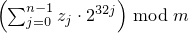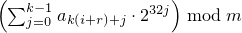# 26 Numerics library [numerics]

## 26.5 Random number generation [rand]

### 26.5.3 Random number engine class templates [rand.eng]

#### 26.5.3.3 Class template subtract_with_carry_engine[rand.eng.sub]

A subtract_with_carry_engine random number engine produces unsigned integer random numbers.

The state xi of a subtract_with_carry_engine object x is of size Ο(r), and consists of a sequence X of r integer values 0 ≤ Xi < m  = 2w; all subscripts applied to X are to be taken modulo r. The state xi additionally consists of an integer c (known as the carry) whose value is either 0 or 1.

The state transition is performed as follows:

1. Let Y = Xi-s - Xi-r - c .

2. Set Xi to y = Y mod m . Set c to 1 if Y < 0, otherwise set c to 0.

Note: This algorithm corresponds to a modular linear function of the form TA(xi) = (a · xi) mod b , where b is of the form mr - ms + 1 and a = b - (b-1) / m .  — end note ]

The generation algorithm is given by GA(xi) = y , where y is the value produced as a result of advancing the engine's state as described above.

template<class UIntType, size_t w, size_t s, size_t r>
class subtract_with_carry_engine{
public:
// types
typedef UIntType result_type;

// engine characteristics
static constexpr size_t word_size = w;
static constexpr size_t short_lag = s;
static constexpr size_t long_lag = r;
static constexpr result_type min() { return 0; }
static constexpr result_type max() { return m - 1; }
static constexpr result_type default_seed = 19780503u;

// constructors and seeding functions
explicit subtract_with_carry_engine(result_type value = default_seed);
template<class Sseq> explicit subtract_with_carry_engine(Sseq& q);
void seed(result_type value = default_seed);
template<class Sseq> void seed(Sseq& q);

// generating functions
result_type operator()();
void discard(unsigned long long z);
};


The following relations shall hold: 0u < s, s < r, 0 < w, and w <= numeric_limits<UIntType>::digits.

The textual representation consists of the values of Xi-r, …, Xi-1, in that order, followed by c.

 explicit subtract_with_carry_engine(result_type value = default_seed); 

Effects: Constructs a subtract_with_carry_engine object. Sets the values of X-r, …, X-1 , in that order, as specified below. If X-1 is then 0, sets c to 1; otherwise sets c to 0.

To set the values Xk, first construct e, a linear_congruential_engine object, as if by the following definition:

linear_congruential_engine<result_type,
40014u,0u,2147483563u> e(value == 0u ? default_seed : value);


Then, to set each Xk, obtain new values z0, …, zn-1 from n = ⌈ w/32 ⌉ successive invocations of e taken modulo 232. Set Xk to.

Complexity: Exactly n · r invocations of e.

 template<class Sseq> explicit subtract_with_carry_engine(Sseq& q); 

Effects: Constructs a subtract_with_carry_engine object. With k = w / 32 and a an array (or equivalent) of length r · k , invokes q.generate(a+0, a+r · k) and then, iteratively for i = -r, …, -1, sets Xi to. If X-1 is then 0, sets c to 1; otherwise sets c to 0.